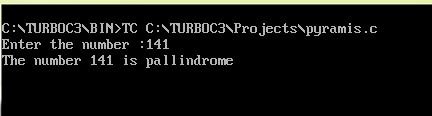UncleCoder.com

UncleCoder.com

Free programming examples and instructions

# C program to Check the number is Palindrome

## C program for how to check the given number is Palindrome

by Krishna

Posted on 27 Jul 2018 Category: C Views: 819

C program to Check a Number is Palindrome or Not

This program reverses an integer entered by the user using for loop. Then, if statement is used to check whether the reversed number is equal to the original number or not. If it is equal, then the entered number is palindrome.

``````#include<stdio.h>
int main(){
int num,r,sum=0,temp;

printf("Enter a number: ");
scanf("%d",&num);

for(temp=num;num!=0;num=num/10){
r=num%10;
sum=sum*10+r;
}
if(temp==sum)
printf("The number %d is a palindrome",temp);
else
printf("%d is not a palindrome",temp);

return 0;
}``````

OUTPUT